## ICSE Physics Previous Year Question Paper 2011 Solved for Class 10

ICSE Paper 2011
PHYSICS

(Two hours)
Answers to this Paper must he written on the paper provided separately.
You will not be allowed to write during the first 15 minutes.
This time is to he spent in reading the question paper.
The time given at the head of this Paper is the time allowed for writing the answers.
Section I is compulsory. Attempt any four questions from Section II.
The intended marks for questions or parts of questions are given in brackets [ ].

SECTION-I (40 Marks)

Attempt all questions from this Section.

Question 1:
(a) (i) Define one newton.
(ii) Write the relation between S.I. unit and C.G.S. unit of force.  

(b) Where does the position of centre of gravity lie for
(i) a circular lamina
(ii) a triangular lamina ?  

(c) A man can open a nut by applying a force of 150 N by using a lever handle of length 0.4 m. What should be the length of the handle if he is able to open it by applying a force of 60 N ?  

(d) Name a machine which can be used to
(i) multiply force
(ii) change the direction of force applied.  

(e) The diagram below shows a lever in use.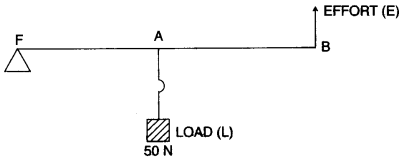(i) To which class of lever does it belong ?
(ii) If FA = 40 cm, AB = 60 cm, then find the mechanical advantage of the lever.  

(a) (i) One Newton is the amount of force required to produce an acceleration of 1 ms-2 in a body of mass 1 kg.
(ii)(b) (i) Centre of the circle or the point of intersection of diameters.
(ii) Centroid or the point of intersection of its medians.

(c) Equating the torque in both the cases :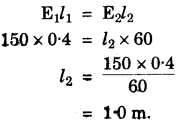(d) (i) Inclined plane
(ii) Pulley

(e) (i) IInd class lever.
(ii)Question 2:
(a) A ball of mass 200 g falls from a height of 5 m. What will be its kinetic energy when it just reaches the ground ? (g = 9.8 ms-2). 

(b) In the diagram below, PQ is a ray of light incident on a rectangular glass block.
(i) Copy the diagram and complete the path of the ray of light through the glass block. In your diagram, mark the angle of incidence by letter ‘i’ and the angle of emergence by the letter ‘e’.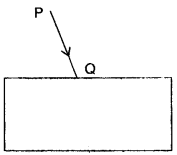(ii) How are the angle ‘i’ and ‘e’ related to each other ? 

(c) A ray of monochromatic light enters a liquid from air as shown in the diagram given below :
(i) Copy the diagram and show in the diagram the path of the ray of light after it strikes the mirror and re-enters the medium of air.
(ii) Mark in your diagram the two angles on the surface of separation when the ray of light moves out from the liquid to air.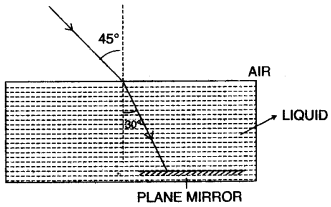(d) (i) When does a ray of light falling on a lens pass through it undeviated ?
(ii) Which lens can produce a real and inverted image of an object ? 

(e) (i) How is the refractive index of a medium related to its real depth and apparent depth ?
(ii) Which characteristic property of light is responsible for the blue colour of the sky ? 

(a) (i)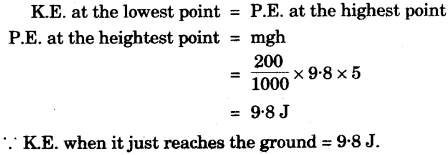(b) (i)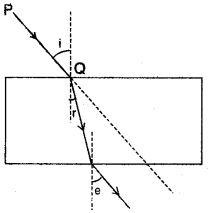(ii) ∠i = ∠e

(c)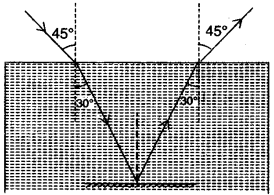(d) (i) When the ray is directed towards the optical centre.
(ii) Convex lens.

(e) (i)(ii) Dispersion of light.

Question 3:
(a) When acoustic resonance takes place a loud sound is heard. Why does this happen ? Explain. 

(b) (i) Three musical instruments give out notes at the frequencies listed below.
Flute : 400 Hz; Guitar : 200 Hz; Trumpet; 500 Hz. Which one of these has the highest pitch ?
(ii) With which of the following frequencies does a tuning fork of 256 Hz resonate ? 288 Hz, 314 Hz, 333 Hz, 512 Hz. 

(c) Two bulbs are marked 100 W, 220 V and 60 W, 110 V. Calculate the ratio of their resistances. 

(d) (i) What is the colour code for the insulation on the earth wire ?
(ii) Write an expression for calculating electrical power in terms of current and resistance. 

(e) Calculate the equivalent resistance between A and B from the following diagram:(a) When acoustic resonance takes place, the natural frequency of vibration of body is equal to the frequency of external force, so the body vibrations of the largest possible amplitude.
Since loudness ∝ Amplitude2.
∴ A loud sound is heard.

(b) (i) Trumpet: 500 Hz (since Pitch ∝ Frequency)
(ii) 512 Hz

(c)(d) (i) Green at Yellow
(ii) P = VI = (IR) I      (∵ By Ohm’s Law, V = IR)
P = I2R

(e) Let R be the equivalent resistance between A and B,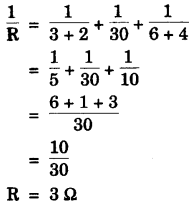Question 4:
(a) Differentiate between heat and temperature.  

(b) (i) Define Calorimetry.
(ii) What is meant by Energy degradation ?  

(c) 200 g of hot water at 80 °C is added to 300 g of cold water at 10 °C. Calculate the final temperature of the mixture of water. Consider the heat taken by the container to be negligible, [specific heat capacity of water is 4200 J kg-1 °C-1]  

(d) Fill in the blanks in the following sentences with appropriate words :
(i) During the emission of a beta particle, the ………. number remains the same.
(ii) The minimum amount of energy required to emit an electron from a metal surface is called ………. .  

(e) A mixture of radioactive substances gives off three types of radiations.
(i) Name the radiation which travels with the speed of light.
(ii) Name the radiation which has the highest ionizing power. 

(a)

 Heat Temperature (i) It is a form of energy (i) It the degree of hotness or codness of a body. (ii) Unit is Joule/Calorie. (ii) Unit is °C, °F or Kelvin.

(b) (i) Heat is a form of energy which flows from the body at a higher temperature to a body at a lower temperature. The measurement of the quantity of heat is called calorimetry.
(ii) The dissipation of energy in the form of non useful energy (usually due to friction) is called Energy degradation.

(c) Let the final temperature be T °C.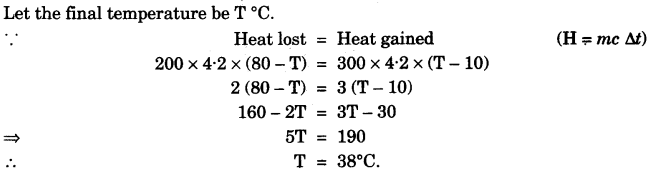(d) (i) Mass
(ii) Work function/Threshold energy

SECTION-II (40 Marks)

Attempt any four questions from this Section.

Question 5:
(a) (i) What is meant by an ideal machine ?
(ii) Write a relationship between the mechanical advantage (M.A.) and velocity ratio (V.R.) of an ideal machine.
(iii) A coolie carrying a load on his head and moving on a frictionless horizontal platform does no work. Explain the reason why. 

(b) Draw a diagram to show the energy changes in an oscillating simple pendulum.
Indicate in your diagram how the total mechanical energy in it remains constant during the oscillation.     

(c) A uniform metre scale can be balanced at the 70.0 cm mark when a mass of 0.05 kg is hung from the 94.0 cm mark.
(i) Draw a diagram of the arrangement.
(ii) Find the mass of the metre scale. 

(a) (i) A machine with 100% efficiency is called an ideal machine.
(ii) For an ideal machine, M.A. = V.R.
(iii) Because the angle between the force applied (vertically upwards) and displacement of load (along the horizontal) is 90°.
∵      W = Fd cos θ
∴      W = Fd cos 90° = 0

(b)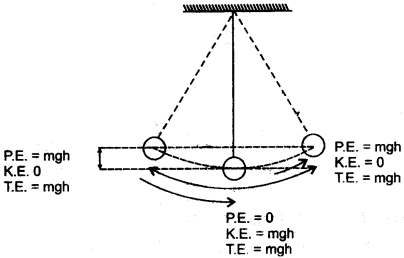(c) (i) See below diagram.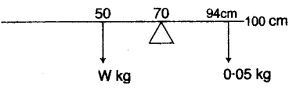(ii)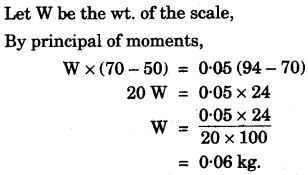Question 6:
(a) (i) State the laws of refraction of light.
(ii) Write a relation between the angle of incidence (i), angle of emergence (e), angle of prism (A) and angle of deviation (d) for a ray of light passing through an equilateral prism.  

(b) (i) Suggest one way, in each case, by which we can detect the presence of:
(ii) Give one use of Infrared radiations.  

(c) An object is placed in front of a lens between its optical centre and the focus and forms a virtual, erect and diminished image.
(i) Name the lens which formed this image.
(ii) Draw a ray diagram to show the formation of the image with the above stated characteristics.  

(a) (i) The laws of refraction of light are :
1. The incident ray, the normal and the refracted ray lie in the same plane.
2. The sine of angle of incidence (i) bears a constant ratio with the sine of angle of refraction (r) for a given pair of medium i.e.,
$$\frac { sin\quad i }{ sin\quad r } =\quad constant$$
(ii) i + e = A + d.

(b) (i) 1. Mercury rises rapidly if a blackened bulb thermometer is kept in these radiations.
2. By the action of silver chloride solution.
(ii) Use of Infrared radiations : Used for therapeutic purposes by doctors.

(c) (i) Concave lens,
(ii)Question 7:
(a) (i) Name the type of waves which are used for sound ranging.
(ii) Why are these waves mentioned in (i) above, not audible to us ?
(iii) Give one use of sound ranging.  

(b) A man standing 25 m away from a wall produces a sound and receives the reflected sound.
(i) Calculate the time after which he receives the reflected sound if the speed of sound in air is 350 ms-1.
(ii) Will the man be able to hear a distinct echo ? Give a reason for your answer. 

(c) (i) Name two safety devices which are connected to the live wire of a household electrical circuit.
(ii) Give one important function of each of these two devices. 

(a) (i) Ultrasonic waves.
(ii) Ultrasonic waves frequency is above 20,000 Hz whereas human audibility range is only till 20,000 Hz.
(iii) Use of sound ranging: Can be used in Radar.

(b) (i)(ii) Yes, because the least time for distinct echo to be heard is 0.1 second.

(c) (1) Fuse and (2) Switch.
(ii) Fuse is used to limit the flow of current. Switch is used to start or stop the flow of current.

Question 8:
(a) (i) Draw a graph of Potential difference (V) versus Current (I) for an ohmic resistor.
(ii) How can you find the resistance of the resistor from this graph ?
(iii) What is a non-ohmic resistor ? 

(b) (i) An electric bulb is marked 100 W, 250 V. What information does this convey ?
(ii) How much current will the bulb draw if connected to a 250 V supply ? 

(c) Three resistors are connected to a 12 V battery as shown in the figure given below: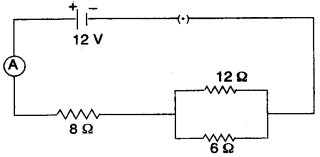(i) What is the current through the 8 ohm resistor ?
(ii) What is the potential difference across the parallel combination of 6 ohm and 12 ohm resistor ?
(iii) What is the current through the 6 ohm resistor ?

(a) (i)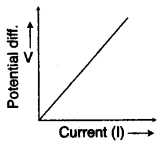(ii) Resistance = Slope of the graph
(iii) A non-ohmic resistor is that for which ratio of potential difference to the current in resistor is not same for all values of potential difference or current. A non-ohmic resistor does not follow Ohm’s Law.

(b) (i) It means that if a bulb is given a 250 V supply, it will consume 100 J of energy per second.
(ii) Let P = VI
⇒   $$I=\frac { 100 }{ 250 }$$
∴ I = 0.4 A

(c)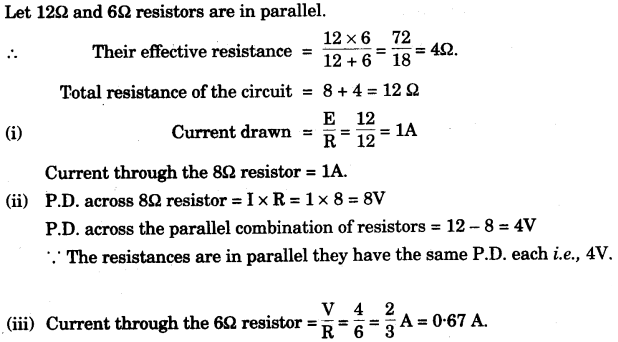Question 9:
(a) (i) Explain why the weather becomes very cold after a hail storm.
(ii) What happens to the heat supplied to a substance when the heat supplied causes no change in the temperature of the substance ?  

(b) (i) When 1 g of ice at 0 °C melts to form lg of water at 0 °C then, is the latent heat absorbed by the ice or given out by it ?
(ii) Give one example where high specific heat capacity of water is used as a heat reservoir.
(iii) Give one example where high specific heat capacity of water is used for cooling purposes. 

(c) 250 g of water at 30 °C is present in a copper vessel of mass 50 g. Calculate the mass of ice required to bring down the temperature of the vessel and its contents to 5 °C.
Specific latent heat of fusion of ice = 336 x 103 J kg-1.
Specific heat capacity of copper vessel = 400 J kg-1 °C-1.
Specific heat capacity of water = 4200 J kg-1 °C-1.   

(a) (i) After the hail storm, ice absorbs heat energy required for melting from the surroundings, so the temperature of the surroundings fall further down and we feel cold.
(ii) Heat is stored as latent heat which is used in the change of state of the substance.

(b) (i) Latent heat of fusion is absorbed by ice.
(ii) Hot water bottles used for fomentation. ,
(iii) Drinks get cooled more quickly by adding pieces of ice at 0°C than the ice cold water at 0°C.

(c) Heat lost by ice = Heat gained by water + Heat gained by copperQuestion 10:
(a) (i) State two properties which a substance should possess when used as a thermionic emitter.
(ii) When an alpha particle gains two electrons it becomes neutral and becomes an atom of an element which is a rare gas. What is the name of this rare gas ?  

(ii) What happens inside the nucleus that causes the emission of beta particle ?
(iii) Express the above change in the form of an equation.  

(c) (i) Name a device which is commonly used to convert an electrical signal into a visual signal.
(ii) The nucleus $$_{ 84 }^{ 202 }{ X }$$ emits an alpha particle and forms the nucleus Y. Represent this change in the form of an equation.
(iii) What changes will take place in the mass number and atomic number of the nucleus Y if it emits gamma radiations ?  

(a) (i) 1. Low Work Function
2. High Melting Point.
(ii) Helium gas.

(b) (i) Radioactivity is a random nuclear phenomenon in which spontaneous emission of α β or γ radiations from the nuclei of atoms during their decay takes place.
(ii) In an unstable nucleus, number of neutrons are more than number of protons. In such a case, a neutron may change to a proton to achieve stability by emitting an electron called beta particle.
(iii)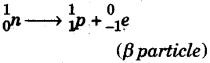(c) (i) Cathode Ray Tube.
(ii)(iii) No change.